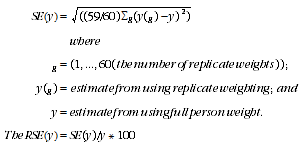4906.0.55.002 - Technical Manual: Personal Safety Survey, Expanded CURF, Australia, 2005
ARCHIVED ISSUE Released at 11:30 AM (CANBERRA TIME) 21/04/2011  Reissue
 Page tools: .mffeedback,#pjs { display :none; } Enable Javascript to Print PagesPrint All RELIABILITY OF ESTIMATES SAMPLE SURVEY ERRORS Two types of error are possible in estimates based on a sample survey: Sampling error occurs because only a small proportion of the total population is used to produce estimates that represent the whole population. Sampling error can be reliably measured as it is calculated based on the scientific methods used to design surveys. Non-sampling error may occur in any data collection, whether it is based on a sample or a full-count (eg Census). Non-sampling error may occur at any stage throughout the survey process, examples include: non-response by selected persons, questions being misunderstood, responses being incorrectly recorded, and errors in coding or processing the survey data. Sampling error Sampling error is the expected difference that could occur between the published estimates, derived from repeated random samples of persons, and the value that would have been produced if all persons in scope of the survey had been included. The magnitude of the sampling error associated with an estimate depends on the sample design, sample size and population variability. One measure of the sampling error for a given estimate is provided by the Standard Error (SE), which is the extent to which an estimate might have varied by chance because only a sample of persons was obtained. Another measure is the Relative Standard Error (RSE), which is the SE expressed as a percentage of the estimate. This measure provides an indication of the percentage errors likely to have occurred due to sampling. Replicate weights and directly calculated standard errors The SEs on estimates from this survey were obtained through the delete-a-group jackknife variance technique. In this technique, the full sample is repeatedly subsampled by successively dropping households from different groups of clusters of households and then the remaining records are reweighted to the survey benchmark population. Through this technique, the effect of the complex survey design and estimation methodology on the accuracy of the survey estimates is stored in the replicate weights. For the 2005 PSS, this process was repeated 60 times to produce 60 replicate weights for each sample unit. The distribution of the 60 replicate estimates based on the full sample estimate is then used to directly calculate the standard error for each full sample estimate. The delete-a-group jackknife method of replicate weighting was used to derive weights, through the following process: 60 replicate groups were formed with each group formed to mirror the overall sample. Units from a Collection District (CD) all belong to the same replicate group and a unit can belong to only one replicate group; one replicate group was dropped from the file and then the remaining records were weighted in the same manner as for the full sample; the records in that group that was dropped received a weight of zero; this process was repeated for each replicate group (i.e. a total of 60 times); and each record had 60 replicate weights attached to it with one of these being the zero weight. Replicate weights enable variances of estimates to be calculated relatively simply. They also enable unit records analyses such as chi-square and logistic regression to be conducted, which take into account the sample design. Replicate weights for any variable of interest can be calculated from the 60 replicate groups, giving 60 replicate estimates. The distribution of this set of replicate estimates, in conjunction with the full sample estimate (based on the general weight) is then used to approximate the variance of the full sample. The Standard Error (SE) for each estimate produced from the CURF can be calculated using the replicate weights provided. When calculating SEs it is important to select the replicate weights which are most appropriate for the analysis being undertaken. For more information see 'Use of weights'. The formula for calculating the Standard Error (SE) and Relative Standard Error (RSE) of an estimate using this technique is shown below.This method can also be used when modelling relationships from unit record data, regardless of the modelling technique used. In modelling, the full sample would be used to estimate the parameter being studied, such as a regression co-efficient, the 60 replicate groups used to provide 60 replicate estimates of the survey parameter. The variance of the estimate of the parameter from the full sample is then approximated, as above, by the variability of the replicate estimates. Use of the delete-a-group jackknife technique for complex estimates, such as regression parameters from a statistical model, is not straightforward and may not be appropriate. The technique described does not apply to investigations where survey weights are not used, such as unweighted statistical modelling. More information on the delete-a-group jackknife technique is provided in the Research Paper: Weighting and Standard Error Estimation for ABS Household Surveys (Methodology Advisory Committee), Jul 1999 (cat. no. 1352.0.55.029). CURF users should be aware that estimates produced from the CURF may differ from those in the published data due to actions taken to preserve confidentiality. Non-sampling error Efforts were made to minimise non-sampling error by careful design and testing of questionnaires, intensive training of interviewers, and extensive editing and quality control procedures at all stages of data processing. However, errors can be made in giving and recording information during an interview. These types of inaccuracies are referred to as non-sampling errors and include errors in the survey scope, response errors, processing errors and bias due to non-response. Document Selection These documents will be presented in a new window.